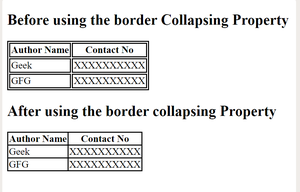Related Articles

# How to remove cellspacing from tables using CSS?

• Last Updated : 01 Dec, 2020

This is a Default behavior of the table cells that there is some space between their Borders. To remove this space we can use the CSS border-collapsing Property. This Property is used to set the borders of the cell present inside the table and tells whether these cells will share a common border or not.

Syntax:

`border-collapse: collapse; `

Example:

 ` ``<``html``> ``    ``<``head``> ``        ``<``title``> ``         ``Remove cellspacing from tables using CSS``        `` ``         ` `        ````        ``<``style``> ``            ``table, td, th { ``                ``border: 2px solid black; ``            ``} ``             ` `            ``#collapseTable { ``                ``border-collapse: collapse; ``            ``} ``        `` ``    `` `` ` `    ``<``body``> ``        ``<``h2``> ``Before using the border Collapsing Property``        `` ``         ` `        ``<``table` `id` `= ``"separateTable"``> ``            ``<``tr``> ``                ``<``th``>Author Name ``                ``<``th``>Contact No ``            `` ``            ``<``tr``> ``                ``<``td``>Geek ``                ``<``td``>XXXXXXXXXX ``            `` ``            ``<``tr``> ``                ``<``td``>GFG ``                ``<``td``>XXXXXXXXXX ``            `` ``        `` ``     ` `        ``<``h2``> ``After using the border collapsing Property``        `` ``         ` `        ``<``table` `id` `= ``"collapseTable"``> ``            ``<``tr``> ``                ``<``th``>Author Name ``                ``<``th``>Contact No ``            `` ``            ``<``tr``> ``                ``<``td``>Geek ``                ``<``td``>XXXXXXXXXX ``            `` ``            ``<``tr``> ``                ``<``td``>GFG ``                ``<``td``>XXXXXXXXXX ``            `` ``        `` ``    `` ```

OutputSupported Browsers are listed below: